# Fei’s teasers for miss A’s ‘Colors’ are everything … FFFFFFFFFFFFFFFFFFFFFFFFFFFFFFWe interrupt your regularly scheduled programming to bring you some hype for the most highly anticipated return since the Book Of Revelations was written — the return of the One True Goddess, Wang Fei Fei.

The holy images, that scholars state are for miss A’s comeback ‘Colors,’ feature the One True Goddess sitting atop a majestic countertop while she examines various mythical elixirs and relics.

—–

Generally, a blog post here will try to accomplish two things. The first is to provide some honest commentary. The second is to lay out that commentary in a somewhat witty and coherent manner.

Usually, those two aims are not opposed to each other.

Unfortunately, this is one of the rare times where they are. This is all I can say about the following Wang Fei Fei teasers.

Ahem.

—–

FFFFFFFFFFFFFFFFFFFFFFFFFFFFFFFFFFFFFFFFFFFFFFFFFFFFFF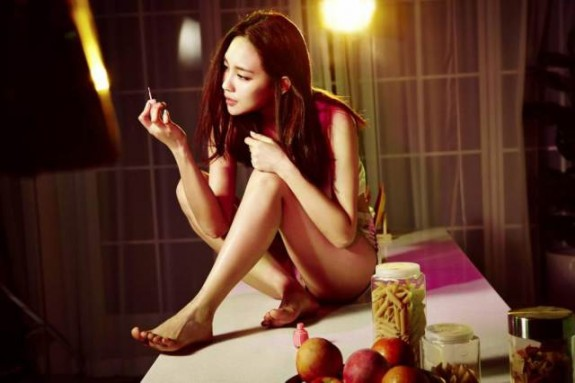FFFFFFFFFFFFFFFFFFFFFFFFFFFFFFFFFFFFFFFFFFFFFFFFFFFFFF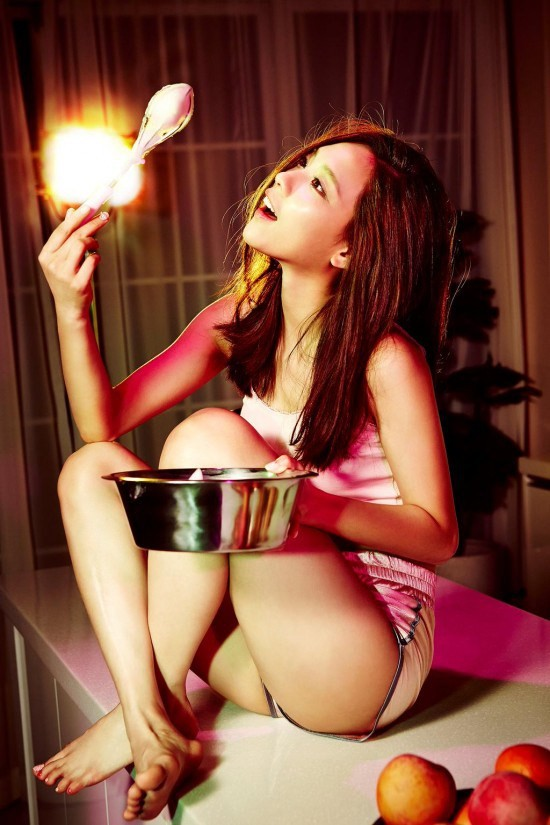FFFFFFFFFFFFFFFFFFFFFFFFFFFFFFFFFFFFFFFFFFFFFFFFFFFFFF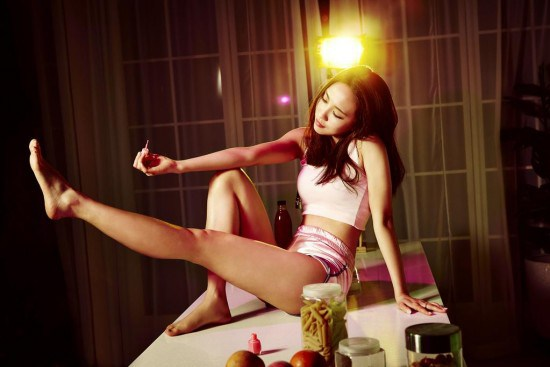FFFFFFFFFFFFFFFFFFFFFFFFFFFFFFFFFFFFFFFFFFFFFFFFFFFFFF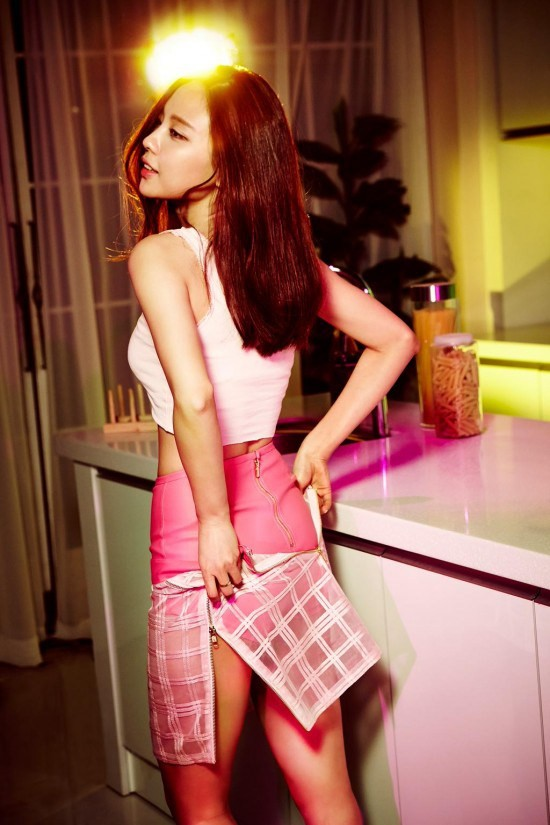*Huff, Huff*

FFFFFFFFFFFFFFFFFFFFFFFFFFFFFFFFFFFFFFFFFFFFFFFFFFFFFF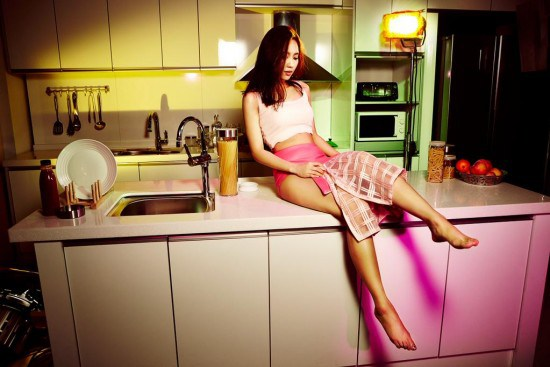FFFFF … *Wheeeeeze* … FFFFFFFFFFFFFFFFFFFFFFFFFFFF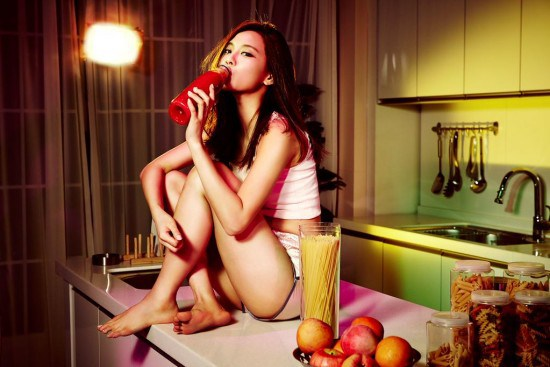FFFFFFFFFFFFFFFF … fffff … fff …

______________________________________